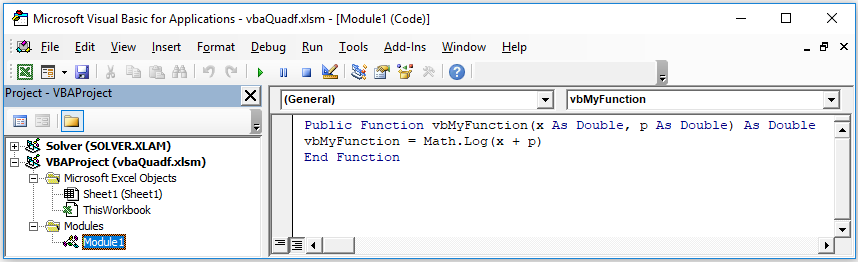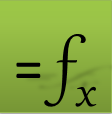# =QUADF(f, x, a, b, [options])

Use QUADF to compute a proper or improper integral using highly accurate adaptive algorithms. With optional arguments, you can override the default integration algorithm as well as supply singular points for the integrand f(x) if applicable.

$\underset{a}{\overset{b}{\int }}f\left(x\right)\cdot dx$

QUADF can be nested to compute multiple integrals of any order.

### Required Inputs

f a reference to the integrand formula.

If your integrand is too complex to represent by formulas, you can code it in a VBA function (see Example 6).

x a reference to the variable of integration.

a the integral lower limit.

b the integral upper limit.

To input infinite limits use the string "INF" or "-INF" (case insensitive).

### Optional Inputs

ctrl a set of key/value pairs for algorithmic control as detailed below.

Description of key/value pairs for algorithmic control

 Key ALGOR Admissible Values (String) QNG,QAG,QAGS,QK15,QK21,QK31,QK41,QK51,QK61 Default Value QAG Remarks If singular points are supplied, the algorithm defaults to internal QAGPIf an infinite limit is specified, the algorithm defaults to internal QAGI
 Keys ATOL, RTOL Admissible Values (Real) >= 0 Default Value ATOL = 1.0E-7, RTOL = 1.0E-5 Remarks Result is reported once either ATOL or RTOL is satisfied.To satisfy ATOL specifically set RTOL to zero and vice versa. ATOL and RTOL cannot be set to zero simultaneously.ATOL and RTOL are not applicable for fixed-order QKn
 Key MAXDIV Admissible Values (Integer) >= 1 Default Value 10000 Remarks Not applicable for algorithm QNG or fixed-order QKn
 Key GKPAIR Admissible Values (Integer) (7-15)(10-21)(15-31)(20-41)(25-51)(30-61) Default Value 2 Remarks Applicable only for algorithm QAG

spts list of known removable singular points for the integrand.

$\underset{1}{\overset{2}{\int }}\frac{2{x}^{5}-x+3}{{x}^{2}}\cdot dx=9-ln2$

### Solution

We define the integrand formula in A1 using X1 as variable. Excel reports the error #DIV/0! for the formula in A1 since X1 is undefined. This error can be ignored since X1 serves only as a dummy variable for the integrand function and its value is irrelevant for the integration computed in A2.

 A 1 #DIV/0! 2 8.306852819

Note for ExceLab 365 users. Define any nonzero value for empty X1 to resolve initial #DIV/0! error in A1 or Excel will not execute dependent formula in A2. Not required for ExceLab 7 or Google Sheets Add-on

$\underset{0}{\overset{1}{\int }}\frac{ln\phantom{\rule{2px}{0ex}}x}{\sqrt{x}}\cdot dx=-4$

### Solution

• We define the integrand formula in A3 using X1 as variable. Excel reports the error #NUM! for the formula in A1 since X1 is undefined. This error can be ignored since X1 serves only as a dummy variable for the integrand function and its value is irrelevant for the integration computed in A4.
• We use QUADF optional parameter number 5 to specify the QAGS algorithm for better accuracy given that there is a singularity at 0.
 A 3 #NUM! 4 -4

Note for ExceLab 365 users. Define any positive value for empty X1 to resolve initial #NUM! error in A3 or Excel will not execute dependent formula in A4. Not required for ExceLab 7 or Google Sheets Add-on

$\underset{0}{\overset{1}{\int }}{|x-\frac{1}{7}|}^{-\frac{1}{7}}\cdot {|x-\frac{2}{3}|}^{-\frac{11}{20}}\cdot dx$

### Solution

The integrand in this example has two singularities at $\frac{1}{7}$ and $\frac{11}{20}$. We make use of optional argument 6 to pass the singular points which are defined in vector B5:B6. Note that we skip over optional argument 5 since we do not alter any defaults.

 A B 5 =ABS(X1-1/7)^(-1/4)*ABS(X1-2/3)^(-11/20) =1/7 6 =QUADF(A5,X1,0,1,,B5:B6) =11/20
 A 5 2.032940662 6 4.253687688

We could pass the singular points in argument 6 using constant array syntax {0.1428571, 0.55}, but we would lose some accuracy rounding 1/7.

$\underset{0}{\overset{\infty }{\int }}\frac{1}{1+{x}^{2}}\cdot dx=\frac{\pi }{2}$

### Solution

 A 7 1 8 1.570796327
$\underset{-\infty }{\overset{\infty }{\int }}{e}^{-{x}^{2}}\cdot dx=\sqrt{\pi }$

### Solution

 A 9 1 10 1.772453851

We demonstrate how to integrate a user defined VBA function with QUADF. You can define your own VBA functions in Excel which is quite powerful when your integrand is difficult to define with standard formulas. We will compute the integral

$\underset{1}{\overset{2}{\int }}log\left(x+p\right)\cdot dx$, where p is a constant parameter.

VBA is supported in ExceLab 7.0 only. ExceLab 365 which is based on cross-platform Office JS technology is not compatible with VBA

### Solution

1. Open Excel and start VBA Editor by pressing Alt+F11
2. Insert a Module from the Insert Tab, then code the following function:3. Save the workbook with the extension xlsm (macro enabled) and close VBA editor
4. In Sheet1, define your integrand formula as shown in A11.We pass the integration variable and any other parameters the VBA function expects.
 A 11 =vbMyFunction(X1, 1) 12 =QUADF(A11,X1,1,2)
 A 11 0 12 0.909542505

Your VBA function name must be prefixed with "vb" to be used with ExceLab solvers.

X1 is just a dummy variable for the integrand function. Its value is ignored.

QUADF algorithms are based on the numerical integration package QUADPACK. QUADF default algorithm is QAG. You can override the default algorithm in optional argument 5 with the key ALGOR For example: =QUADF(f, x, a, b, {"algor","qags"}).

QNG
A non-adaptive algorithm which uses fixed Gauss-Kronrod-Patterson abscissae to sample the integrand at a maximum of 87 points. It is suitable for fast integration of smooth functions.
QAG
An adaptive integration algorithm which divides the integration region into subintervals, and on each iteration the subinterval with the largest estimated error is bisected. This reduces the overall error rapidly, as the subintervals become concentrated around local difficulties in the integrand. The integration rule can be set by the GKPAIR key/value pair.
QAGS
Combines QAG with the Wynn epsilon-algorithm to speed up the integration of many types of integrable singularities. It uses 21-point Gauss-Kronrod rule.
QAGP
Applies QAGS taking into account user-supplied locations of singular points.
QAGI
Used for improper integrals. The integral is mapped onto the semi-open interval (0,1] using the transformation x = (1-t)/t. It is then integrated using the QAGS algorithm using a 15-point Gauss-Kronrod rule
QKn
The fixed-order Gauss-Legendre integration routines are provided for fast integration of smooth functions. The n-point Gauss-Legendre rule is exact for polynomials of order 2*n-1 or less. Rules are available for n = 15, 21, 31, 41, 51, 61. (e.g., QK21.)
Question or Comment? Email us:
support @ excel-works.comExceLab: Transforming Excel into a Calculus Power House

ExceLab functions and methods are protected by USA Patents 10628634, 10114812, 9892108 and 9286286.